### Binomial• (a.) Having two names; -- used of the system by which every animal and plant receives two names, the one indicating the genus, the other the species, to which it belongs. • (n.) An expression consisting of two terms connected by the sign plus (+) or minus (-); as, a + b, or 7 - 3. • (a.) Consisting of two terms; pertaining to binom...
Found on http://thinkexist.com/dictionary/meaning/binomial/

### binomial<mathematics> An expression consisting of two terms connected by the sign plus (+) or minus; as, a+b, or 7-3. ... Origin: L. Bis twice + nomen name: cf. F. Binome, LL. Binomius (or fr. Bi- + Gr. Distribution ?). Cf. Monomial. ... 1. Consisting of two terms; pertaining to binomials; as, a binomial root. ... 2. Having two names; used of the sys...
Found on http://www.encyclo.co.uk/local/20973

### binomial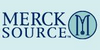(bi-no´me-әl) composed of two terms, such as names of organisms that are formed by combination of genus and species names.
Found on http://www.encyclo.co.uk/local/21001

### binomialadjective relating to binomials; `binomial expression`
Found on https://www.encyclo.co.uk/local/20974

### BinomialBi·no'mi·al noun [ Latin bis twice + nomen name: confer French binome , Late Latin binomius (or from bi- + Greek ... distribution ?). Confer Monomial .] (Alg.) An expression consisting of two terms connected by the sign plus (+) or minus (-)...
Found on http://www.encyclo.co.uk/webster/B/53

### Binomial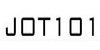A mathematical expression is binomial when it is concerned with the difference or the sum of two terms
Found on http://jot101.com/2015/05/a-z-of-science-fiction-words/

### Binomial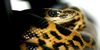a scientific name comprised of two parts genus and species. Ex. Crotalus adamanteus. Crotalus is the genus for Rattlesnakes and adamanteus is the species name for the Eastern Diamondback Rattlesnake.
Found on http://www.kingsnake.com/articles/Glossary.html

### Binomial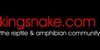a scientific name comprised of two parts, genus and species. Ex. Crotalus adamanteus. Crotalus is the genus for Rattlesnakes and adamanteus is the species name for the Eastern Diamondback Rattlesnake.
Found on http://www.kingsnake.com/articles/Glossary.html

### binomial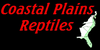A scientific name consisting of two parts, a genus and a species
Found on http://www.coastalplainsreptiles.com/glossary.htm

### binomialAn polynomial expression containing two terms, joined by + or -. The binomial theorem gives the result of raising a binomial expression to a power; the expansion and the series it leads to are called the binomial expansion and the binomial series. A binomial distribution is described by a formula re...
Found on http://www.daviddarling.info/encyclopedia/B/binomial.html

### binomial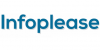binomial (bī"nō'mēul) , polynomial expression (see polynomial) containing two terms, for example, x+y. The binomial theorem, or binomial formula, gives the expansion of the nth power of a binomial (x+y) for n=1, 2, 3, … , as follows:(x+y)n = xn + (n/1)xn-1y + n(n-1)/(...

### binomial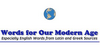binomial, binomialism 1. Consisting of two terms; a mathematical expression made up of two terms and a plus or minus sign. 2. In biology, a pair of Latin or Latinized words forming a scientific name in the classification of plants, animals, and microorganisms. The first word represents the genus and the second the species.
Found on http://www.wordinfo.info/words/index/info/view_unit/2560/

### binomial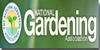Consisting of two names; for example, a botanical name consists of the genus name followed by the species epithet.
Found on http://assoc.garden.org/courseweb/course1/glossary.htm

### Binomial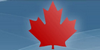consisting of two parts

### binomial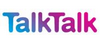In mathematics, an expression consisting of two terms, such as a + b or a - b
Found on http://www.talktalk.co.uk/reference/encyclopaedia/hutchinson/m0000358.html

### binomial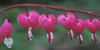making use of names consisting of two words to form the scientific name (or combination) in a Latin form. For example, where the first is the name of the genus to which the species belongs, and the second is the epithet given to that species to distinguish it from others in the same genus.
Found on http://en.wikipedia.org/wiki/Glossary_of_botanical_terms

### binomial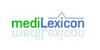Type: Term Pronunciation: bī-nō′mē-ăl Definitions: 1. A set of two terms or names; in the probabilistic or statistical sense it corresponds to a Bernoulli trial.
Found on http://www.medilexicon.com/medicaldictionary.php?t=10237

### binomial[adj] - consisting of two names or terms 2. [adj] - relating to binomials 3. [n] - a quantity expressed as a sum or difference of two terms
Found on http://www.webdictionary.co.uk/definition.php?query=binomial
No exact match found